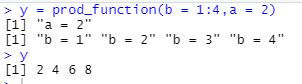top of page
Search
• Ekta Aggarwal

# User Defined functions in R

When you need to do some computations multiple times, instead of writing the same code N number of times, a good practise is to write the code chunk once as a function and then call the function with a single line of code.

In this tutorial, we shall be covering all the aspects of creating a user defined function (UDF) in R.

### Syntax:

function_name = function(parameter1,paramater2, ...) {

Do Something

}

Providing parameters in a function are optional.

## Creating our first function:

A function can be used to print the statements. In the following code we are creating a function my_first_function which prints the statements.

```my_first_function = function(){
print("This is my first function!")
}```

### Calling a function

To get the output of a function we need to call it as follows:

`my_first_function()`## Function inside a for loop

Sometimes we need to call the function repeatedly in a for loop.. For this we define the function outside the for loop and it is being called inside the for loop as follows:

```for(i in 1:5){
my_first_function()
}```## Functions returning an output

Functions also have the power to return an output.

Here, we are creating a function prod_function which takes two parameters : a and b, prints their values and returns their product as an output.

If we want functions to return an output we must use return statement.

```prod_function = function(a,b){
print(paste0("a = ",a))
print(paste0("b = ",b))
return(a*b)
}

y =prod_function(2,3)
print(y)```Parameters take value in the order as they appear. In the following code, a takes values 1 to 4, while b = 2.

```y = prod_function(1:4,2)
y```### Switching the order of parameters:

If you want to switch the order of parameters then while calling the function you need to specify the names of parameters with the values.

In the following code, b takes values 1 to 4, while a = 2.

```y = prod_function(b = 1:4,a = 2)
y```## The ... parameter in a function:

In the following function, we are calculating mean of a vector:

```mean_function  = function(x){
return(mean(x))
}
abc = 1:10
mean_function(abc)```

What if our vector contains an NA? Our output would be NA.

```def = c(1:10,NA)
mean_function(def)```

If we want to provide extra parameters while calling a function we need to specify ... parameter.

Here it means we can provide na.rm = T (which is our parameters in mean function) while calling our UDF.

```mean_function  = function(x,...){
return(mean(x,...))
}
mean_function(def,na.rm = T)```See All
bottom of page